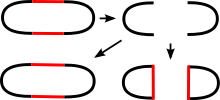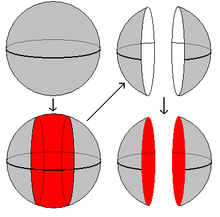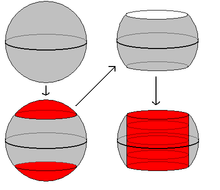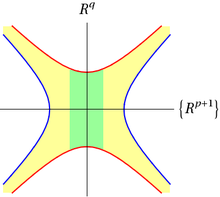# Surgery theory

Surgery theory

In mathematics, specifically in geometric topology, surgery theory is a collection of techniques used to produce one manifold from another in a 'controlled' way, introduced by Milnor (1961). Surgery refers to cutting out parts of the manifold and replacing it with a part of another manifold, matching up along the cut or boundary. This is closely related to, but not identical with, handlebody decompositions. It is a major tool in the study and classification of manifolds of dimension greater than 4.

More technically, the idea is to start with a well-understood manifold M and perform surgery on it to produce a manifold M' having some desired property, in such a way that the effects on the homology, homotopy groups, or other interesting invariants of the manifold are known.

The classification of exotic spheres by Kervaire and Milnor (1963) led to the emergence of surgery theory as a major tool in high-dimensional topology.

## Surgery on a manifold

Recall that in general, if X, Y are manifolds with boundary, then the boundary of the product manifold is$\partial(X \times Y)=(\partial X \times Y)\cup(X \times \partial Y)$. The basic observation which justifies surgery is that the space$S^p \times S^{q-1}$ can be understood either as the boundary of$D^{p+1} \times S^{q-1}$ or as the boundary of$S^p \times D^q$. In symbols,$\partial(S^p \times D^q)=S^p \times S^{q-1}=\partial(D^{p+1}\times S^{q-1})$.

Now, given a manifold M of dimension n = p + q and an embedding$\phi\colon S^p\times D^q\to M$, define another n-dimensional manifold M' to be$M':= (M-\operatorname{int~im}\phi)\;\cup_{\phi|_{S^p\times S^{q-1}}} (D^{p+1} \!\times\! S^{q-1}).$

One says that the manifold M' is produced by a surgery cutting out$S^p \times D^q$ and gluing in$D^{p+1} \times S^{q-1}$, or by a p-surgery if one wants to specify the number p. Strictly speaking, M' is a manifold with corners, but there is a canonical way to smooth them out. Notice that the submanifold that was replaced in M was of the same dimension as M (it was of codimension 0).

Surgery is closely related to (but not the same as) handle attaching. Given an (n + 1)-manifold with boundary$(L,\partial L)$ and an embedding$\phi\colon S^p\times D^q\to \partial L$, where n = p + q, define another (n + 1)-manifold with boundary L' by$L':= L\; \cup_\phi (D^{p+1}\!\!\times\!D^q).$

The manifold L' is obtained by attaching a (p + 1)-handle, with$\partial L'$ obtained from$\partial L$ by a p-surgery$\partial L'= (\partial L-\operatorname{int~im}\phi)\; \cup_{\phi|_{S^p\times S^{q-1}}} (D^{p+1} \!\times\! S^{q-1}).$

A surgery on M not only produces a new manifold M', but also a cobordism W between M and M'. The trace of the surgery is the cobordism (W;M,M'), with$W:= (M\times I)\; \cup_{S^p\times D^q\times \{1\}} (D^{p+1}\!\times\!D^q)$

the (n + 1)-dimensional manifold with boundary$\partial W=M \cup M'$ obtained from the product$M \times I$ by attaching a (p + 1)-handle$D^{p+1} \times D^q$.

Surgery is symmetric in the sense that the manifold M can be re-obtained from M' by a (q − 1)-surgery, the trace of which coincides with the trace of the original surgery, up to orientation.

In most applications, the manifold M comes with additional geometric structure, such as a map to some reference space, or additional bundle data. One then wants the surgery process to endow M' with the same kind of additional structure. For instance, a standard tool in surgery theory is surgery on normal maps: such a process changes a normal map to another normal map within the same bordism class.

### Examples

1. Surgery on the circleFig. 1

As per the above definition, a surgery on the circle consists of cutting out a copy of$S^0 \times D^1$ and gluing in$S^0 \times D^1$. The pictures in Fig. 1 show that the result of doing this is either (i) S1 again, or (ii) two copies of S1.Fig. 2aFig. 2b

2. Surgery on the 2-sphere

In this case there are more possibilities, since we can start by cutting out either$S^1 \times D^1$ or$S^0 \times D^2$.

• (a)$S^1 \times D^1$: If we remove a cylinder from the 2-sphere, we are left with two disks. We have to glue back in$S^0 \times D^2$ - that is, two disks - and it's clear that the result of doing so is to give us two disjoint spheres. (Fig. 2a)Fig. 2c. This shape cannot be embedded in 3-space.
• (b)$S^0 \times D^2$: Having cut out two disks$S^0 \times D^2$, we glue back in the cylinder$S^1 \times D^1$. Interestingly, there are two possible outcomes, depending on whether our glueing maps have the same or opposite orientation on the two boundary circles. If the orientations are the same (Fig. 2b), the resulting manifold is the torus$S^1 \times S^1$, but if they are different, we obtain the Klein Bottle (Fig. 2c).

3. Surgery on the n-sphere

If n = p + q, then$S^n=\partial D^{n+1}\approx \partial (D^{p+1}\times D^q)= S^p\times D^q\;\cup\;D^{p+1}\times S^{q-1}$. The p-surgery on Sn is therefore$D^{p+1}\times S^{q-1}\;\cup\;D^{p+1}\times S^{q-1}=S^{p+1}\times S^{q-1}$. Examples 1 and 2 above were a special case of this.

4. Morse functions

Suppose that f is a Morse function on an (n + 1)-dimensional manifold, and suppose that c is a critical value with exactly one critical point in its preimage. If the index of this critical point is p + 1, then the level-set$M':=f^{-1}(c+\epsilon)$ is obtained from$M:=f^{-1}(c-\epsilon)$ by a p-surgery. The bordism$W:=f^{-1}([c-\epsilon,c+\epsilon])$ can be identified with the trace of this surgery.Fig. 4

Indeed, in some coordinate chart around the critical point, the function f is of the form$-\Vert x\Vert^2 + \Vert y\Vert^2$, with$x\in R^{p+1}, y\in R^q$, and p + q + 1 = n + 1. Fig. 3 shows, in this local chart, the manifold M in blue and the manifold M' in red. The colored region between M and M' corresponds to the bordism W. The picture shows that W is diffeomorphic to the union$W \cong M\times I \cup_{S^p\times D^q} D^{p+1}\times D^q$

(neglecting the issue of straightening corners), where$M\times I$ is colored in yellow, and$D^{p+1}\times D^q$ is colored in green. The manifold M', being a boundary component of W, is therefore obtained from M by a p-surgery.

Since every bordism between closed manifolds has a Morse function where different critical points have different critical values, this shows that any bordism can be decomposed into traces of surgeries (handlebody decomposition). In particular, every manifold M may be regarded as a bordism from the boundary$\partial M$ (which may be empty) to the empty manifold, and so may be obtained from$\partial M \times I$ by attaching handles.

### Effects on homotopy groups, and comparison to cell-attachment

Intuitively, the process of surgery is the manifold analog of attaching a cell to a topological space, where the embedding ϕ takes the place of the attaching map. A simple attachment of a (q + 1)-cell to an n-manifold would destroy the manifold structure for dimension reasons, so it has to be thickened by crossing with another cell.

Up to homotopy, the process of surgery on an embedding$\phi\colon S^p\times D^q\to M$ can be described as the attaching of a (p + 1)-cell, giving the homotopy type of the trace, and the detaching of a q-cell to obtain N. The necessity of the detaching process can be understood as an effect of Poincaré duality.

In the same way as a cell can be attached to a space to kill an element in some homotopy group of the space, a p-surgery on a manifold M can often be used to kill an element$\alpha\in\pi_p(M)$. Two points are important however: Firstly, the element$\alpha\in\pi_p(M)$ has to be representable by an embedding$\phi\colon S^p\times D^q\to M$ (which means embedding the corresponding sphere with a trivial normal bundle). For instance, it is not possible to perform surgery on an orientation-reversing loop. Secondly, the effect of the detaching process has to be considered, since it might also have an effect on the homotopy group under consideration. Roughly speaking, this second point is only important when p is at least of the order of half the dimension of M.

## Application to classification of manifolds

The origin and main application of surgery theory lies in the classification of manifolds of dimension greater than four. Loosely, the organizing questions of surgery theory are:

• Is X a manifold?
• Is f a diffeomorphism?

More formally, one must ask whether up to homotopy:

• Does a space X have the homotopy type of a smooth manifold?
• Is a homotopy equivalence$f\colon M \to N$ between two smooth manifolds homotopic to a diffeomorphism?

It turns out that the second ("uniqueness") question is a relative version of a question of the first ("existence") type; thus both questions can be treated with the same methods.

Note that surgery theory does not give a complete set of invariants to these questions. Instead, it is obstruction-theoretic: there is a primary obstruction, and a secondary obstruction called the surgery obstruction which is only defined if the primary obstruction vanishes, and which depends on the choice made in verifying that the primary obstruction vanishes.

### The surgery approach

In the classical approach, as developed by Browder, Novikov, Sullivan and Wall, surgery is done on normal maps of degree one. Using surgery, the question "Is the normal map$f\colon M\to X$ of degree one cobordant to a homotopy equivalence?" can be translated (in dimensions greater than four) to an algebraic statement about some element in an L-group of the group ring Z1(X)]. More precisely, the question has a positive answer if and only if the surgery obstruction$\sigma(f)\in L_n(Z[\pi_1 X])$ is zero, where n is the dimension of M.

For example, consider the case where the dimension n=4k is a multiple of four, and π1(X) = 0. It is known that L4k(Z) is isomorphic to Z; under this isomorphism the surgery obstruction of f maps, up to a scalar factor, to the difference of the signatures σ(X) − σ(M) of X and M. Hence a normal map of degree one is cobordant to a homotopy equivalence if and only if the signatures of domain and codomain agree.

Coming back to the "existence" question from above, we see that a space X has the homotopy type of a smooth manifold if and only if it receives a normal map of degree one whose surgery obstruction vanishes. This leads to a multi-step obstruction process: In order to speak of normal maps, X must satisfy an appropriate version of Poincaré duality which turns it into a Poincaré complex. Supposing that X is a Poincaré complex, the Pontryagin-Thom construction shows that a normal map of degree one to X exists if and only if the Spivak normal fibration of X has a reduction to a stable vector bundle. If normal maps of degree one to X exist, their bordism classes (called normal invariants) are classified by the set of homotopy classes [X,G / O]. Each of these normal invariants has a surgery obstruction; X has the homotopy type of a smooth manifold if and only if one of these obstructions is zero. Stated differently, this means that there is a choice of normal invariant with zero image under the surgery obstruction map$[X, G/O] \to L_n(Z[\pi_1(X)]).$

### Structure sets and surgery exact sequence

The concept of structure set is the unifying framework for both questions of existence and uniqueness. Roughly speaking, the structure set of a space X consists of homotopy equivalences$M\to X$ from some manifold to X, where two maps are identified under a bordism-type relation. A necessary (but not in general sufficient) condition for the structure set of a space X to be non-empty is that X be an n-dimensional Poincaré complex, i.e. that the homology and cohomology groups be related by isomorphisms$H^*(X) \cong H_{n-*}(X)$ of an n-dimensional manifold, for some integer n. Depending on the precise definition and the category of manifolds (smooth, PL, or topological), there are various versions of structure sets. Since, by the s-cobordism theorem, certain bordisms between manifolds are isomorphic (in the respective category) to cylinders, the concept of structure set allows a classification even up to diffeomorphism.

The structure set and the surgery obstruction map are brought together in the surgery exact sequence. This sequence allows to determine the structure set of a Poincaré complex once the surgery obstruction map (and a relative version of it) are understood. In important cases, the smooth or topological structure set can be computed by means of the surgery exact sequence. Examples are the classification of exotic spheres, and the proofs of the Borel conjecture for negatively curved manifolds and manifolds with hyperbolic fundamental group.

In the topological category, the surgery exact sequence is the long exact sequence induced by a fibration sequence of spectra. This implies that all the sets involved in the sequence are in fact abelian groups. On the spectrum level, the surgery obstruction map is an assembly map whose fiber is the block structure space of the corresponding manifold.

Wikimedia Foundation. 2010.

### Look at other dictionaries:

• Surgery (disambiguation) — Surgery is a medical specialty that uses operative treatment. It also refers to the place where surgery is performed, or the offices of the practitioner, the surgeon.Surgery may also mean:In medicine: * In American English, a surgical procedure… …   Wikipedia

• surgery — /serr jeuh ree/, n., pl. surgeries for 3 5. 1. the art, practice, or work of treating diseases, injuries, or deformities by manual or operative procedures. 2. the branch of medicine concerned with such treatment. 3. treatment, as an operation,… …   Universalium

• Obstruction theory — In mathematics, obstruction theory is a name given to two different mathematical theories, both of which yield cohomological invariants. Contents 1 In homotopy theory 2 In geometric topology 3 In surgery theory …   Wikipedia

• L-theory — Algebraic L theory is the K theory of quadratic forms; the term was coined by C. T. C. Wall, with L being used as the letter after K . Algebraic L theory (also known as hermitian K theory )is very important in surgery theory. DefinitionOne can… …   Wikipedia

• Algebraic K-theory — In mathematics, algebraic K theory is an important part of homological algebra concerned with defining and applying a sequence Kn(R) of functors from rings to abelian groups, for all integers n. For historical reasons, the lower K groups K0 and… …   Wikipedia

• Dehn surgery — In topology, a branch of mathematics, a Dehn surgery, named after Max Dehn, is a specific construction used to modify 3 manifolds. The process takes as input a 3 manifold together with a link. Dehn surgery can be thought of as a two stage process …   Wikipedia

• K-theory — In mathematics, K theory is a tool used in several disciplines. In algebraic topology, it is an extraordinary cohomology theory known as topological K theory. In algebra and algebraic geometry, it is referred to as algebraic K theory. It also has …   Wikipedia

• History of surgery — Surgery is the branch of medicine that deals with the physical manipulation of a bodily structure in order to diagnosis, prevent or cure an ailment. Ambroise Paré, a 16th century French surgeon stated that there were five reasons to perform… …   Wikipedia

• Migraine surgery — is any surgical operation undertaken with the goal of reducing or preventing migraines. Innovative surgical techniques have been developed to help patients with migraine headaches. Migraines affect an estimated 10% of the worldwide population… …   Wikipedia

• Small cancellation theory — In the mathematical subject of group theory, small cancellation theory studies groups given by group presentations satisfying small cancellation conditions, that is where defining relations have small overlaps with each other. It turns out that… …   Wikipedia